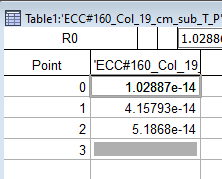# Converting waves into multiple wavesGood morning the amazing Igor community,

I have a simple wave created within a macro that has 3 points. I would like to write a line where I can kind of "transpose" those three points and make each of them a point where I can call it something like "treatment A", "treatment B", "treatment C"

do you have any ideas how to do that?

Thank you very much in advance and attached you can see the picture of a created wave

All the bestHi,

If I understand correctly, you'd like three waves each with one point?

// an example wave
Make/O/N=(3) myWave = gnoise(1)
// show it
edit myWave
// transpose it
MatrixTranspose myWave
// generate the three waves
SplitWave/NAME=("treatmentA;treatmentB;treatmentC") myWave
// have a look at them
edit treatmentA,treatmentB,treatmentC

In reply to by sjr51

Thank you very much!! That is exactly what I want. The script runs well until the line:

SplitWave/NAME=("treatmentA;treatmentB;treatmentC") myWave

It tells me "name already exists as a wave" and it is referring to "myWave" at the end of this line, any ideas what to change?

I have added the overwrting option and I went through the error. Now I have another problem of not being able to change the name of the columns (kindly see the attached picture)

Please not that to create such a wave, I have \$ during creation of the wave. I have also included the full macro that I am using. In this case the \$IR produced wave is the one I want to transpose and relabel it

#pragma TextEncoding = "UTF-8"
#pragma rtGlobals=3     // Use modern global access method and strict wave access.

Macro poolanalysisfixFinal(EndStr)

String Endstr="sub"
prompt Endstr, "Enter match string here"

string  traceslist, currenttracename, dep, depolarization, IR
traceslist = Wavelist("*"+Endstr,";","")

variable traceindex=0

do
currenttracename = StringFromList (traceindex, traceslist,  ";")
if (strlen(currenttracename) == 0)
break
endif

dep=currenttracename+"dep"
duplicate/O \$currenttracename \$dep
depolarization=currenttracename+"_T"

Make/O/N=11 \$depolarization
\$depolarization = mean(\$dep,pnt2x(\$dep,5),pnt2x(\$dep,302)) //baseline
\$depolarization = mean(\$dep,pnt2x(\$dep,328),pnt2x(\$dep,625)) //first depolarization
\$depolarization = mean(\$dep,pnt2x(\$dep,651),pnt2x(\$dep,948))  //second depolarization
\$depolarization = mean(\$dep,pnt2x(\$dep,974),pnt2x(\$dep,1271))  //third depolarization
\$depolarization = mean(\$dep,pnt2x(\$dep,1297),pnt2x(\$dep,1594)) //fourth depolarization
\$depolarization = mean(\$dep,pnt2x(\$dep,1620),pnt2x(\$dep,1917)) //fifth depolarization
\$depolarization = mean(\$dep,pnt2x(\$dep,1943),pnt2x(\$dep,2240))  //sixth depolarization
\$depolarization = mean(\$dep,pnt2x(\$dep,2359),pnt2x(\$dep,2656))  //seventh depolarization
\$depolarization = mean(\$dep,pnt2x(\$dep,2775),pnt2x(\$dep,3072))  //eighth depolarization
\$depolarization = mean(\$dep,pnt2x(\$dep,3191),pnt2x(\$dep,3488))  //ninth depolarization
\$depolarization = mean(\$dep,pnt2x(\$dep,3607),pnt2x(\$dep,3904))  //tenth depolarization

//making a wave to take the 6th and the 10th points corresponding to either IRP or RRPtotal
//duplicate/O \$currenttracename IRpools
IR=currenttracename+"_I"
Make/O/N=3 \$IR

\$IR= \$depolarization
\$IR= \$depolarization-\$depolarization
\$IR= \$depolarization

MatrixTranspose \$IR
splitWave/O/NAME=("IRP;RRP;RRPtotal") \$IR
//Killwaves \$IR

//\$PA=3

//calculating DCM for each depolarization step
//\$depolarization=0
\$depolarization=\$depolarization-\$depolarization
\$depolarization =\$depolarization-\$depolarization
\$depolarization =\$depolarization-\$depolarization
\$depolarization =\$depolarization-\$depolarization
\$depolarization =\$depolarization-\$depolarization
\$depolarization =\$depolarization-\$depolarization
\$depolarization =\$depolarization-\$depolarization
\$depolarization =\$depolarization-\$depolarization
\$depolarization =\$depolarization-\$depolarization
\$depolarization =\$depolarization-\$depolarization

traceindex+=1

print "traceindex = ",traceindex
print currenttracename
display \$depolarization

while(1)

KillAllGraphs()

endmacro

Function KillAllGraphs()
string fulllist = WinList("*", ";","WIN:1")
string name, cmd
variable i
for(i=0; i<itemsinlist(fulllist); i +=1)
name= stringfromlist(i, fulllist)
sprintf  cmd, "Dowindow/K %s", name
execute cmd
endfor
end

First, I missed a terminating semicolon in my example, it should have said:

`SplitWave/NAME=("treatmentA;treatmentB;treatmentC;")/O myWave`

And yes, overwriting is good, but as you have discovered the names are fixed so that they will continually overwrite themselves in your do-while loop. So what you need to do is construct a string of semicolon separated names in each iteration. Like you do for the IR string variable. Here's one way:

// outside loop
String expr = ""

// inside your loop
IR=currenttracename+"_I"
Make/O/N=3 \$IR
\$IR= \$depolarization
\$IR= \$depolarization-\$depolarization
\$IR= \$depolarization

expr = currenttracename + "_IRP;" + currenttracename + "_RRP;" + currenttracename + "_RRPtotal;"
MatrixTranspose \$IR
SplitWave/O/NAME=expr \$IR

In reply to by sjr51

Dear sjr51,

Fantastic!! Thank you very much for the great help. That just leaves me with one thing; I get the IRP, RRP, and RRPtotal in three seperate waves and not in a single one with multiple columns. Is that how I should do it? or is there a way where I can fuse the three in the same wave as I was intending ?

Many Many thanks!!

Perhaps sjr51 misinterpreted what you are trying to do. It seems to me that you want to end up with 1 wave that has 1 row and 3 columns, and each column has the dimension label set. If that's what you want, it's pretty easy to do. Note, however, that there may be no reason to make the wave 1 row by 3 columns. You could also make the wave a 1D wave with 3 rows.

Here is an example function. I recommend that you run one line at a time on the command line so you can see what is happening.

Function test()
// Creating this wave based on the picture
Make/O/N=(3)/D wave1 = {1.02887e-14,4.15793e-14,5.1868e-14}

Edit wave1.ld   // At this point we haven't set the dimension labels

// Set the dimension labels
SetDimLabel 0, 0, treatmentA, wave1
SetDimLabel 0, 1, treatmentB, wave1
SetDimLabel 0, 2, treatmentC, wave1

// Example of how to use dimension labels
// with a 1D wave
print wave1[%treatmentB]

// At this point you have a 1D wave with 3 rows.
// Maybe that's all you need?
// If not, the next command makes
// it a 2D wave with 1 row and 3 columns.
MatrixTranspose wave1

// Example of how to use dimension labels
// with a 2D wave
print wave1[%treatmentB]
End

As a note, if you knew from the beginning that you wanted to create a 2D wave with 1 row and 3 columns, your make statement would be:

`Make/O/N=(1,3)/D wave1`

Hello,

It's not clear what form you want the final three values to be in.  Do you want them in a wave where you can refer to them by some name ("IRP, RRP, RRPtotal")?  In that case look into row (or column) labels.  Type this in the command line.

`displayhelptopic "SetDimLabel"`

Or the values could be saved as variables (globals if you need them to persist when your function execution ends):

Variable IRP = \$depolarization
Variable RRP = \$depolarization-\$depolarization
Variable RRPtotal = \$depolarization